## Hypervalent or not? A fluxional triselenide.

Another post inspired by a comment on an earlier one; I had been discussing compounds of the type I.In (n=4,6) as possible candidates for hypervalency. The comment suggests the below as a similar analogue, deriving from observations made in 1989.This compound was investigated using 77Se NMR, with the following conclusions:

1. The compound is fluxional, with the lines at room temperature broadened compared to those at -50°C.
2. At -50°C the peaks are sharp enough to discern 1JSe-Se couplings, with multiplicities and integrations that suggest a central Se is surrounded by four equivalent further Se atoms, with shifts of 655.1 and 251.2 ppm.
3. The magnitude of this 1JSe-Se coupling (391 Hz) leads to the suggestion of a considerable contribution of a resonance form with Se=Se bonds (structure 2 above).
4. This was supported by 2J13C-77Se couplings which also imply a symmetrically coordinated central  Se.
5. Thus the two resonance forms 1 or 2 above were suggested as the predominant form at -50°C, with an increasing incursion of the open chain isomer 3 at higher temperatures giving rise to the observed fluxional dynamic behaviour.
6. One may surmise from these results that the central Se is certainly hypercoordinated and by the classical interpretations hypervalent.

Here are some calculations (R=H), at the ωB97XD/Def2-TZVPP/SCRF=chloroform level.‡ In red are the calculated Wiberg Se-Se bond orders, which give little indication of any Se=Se double bond character.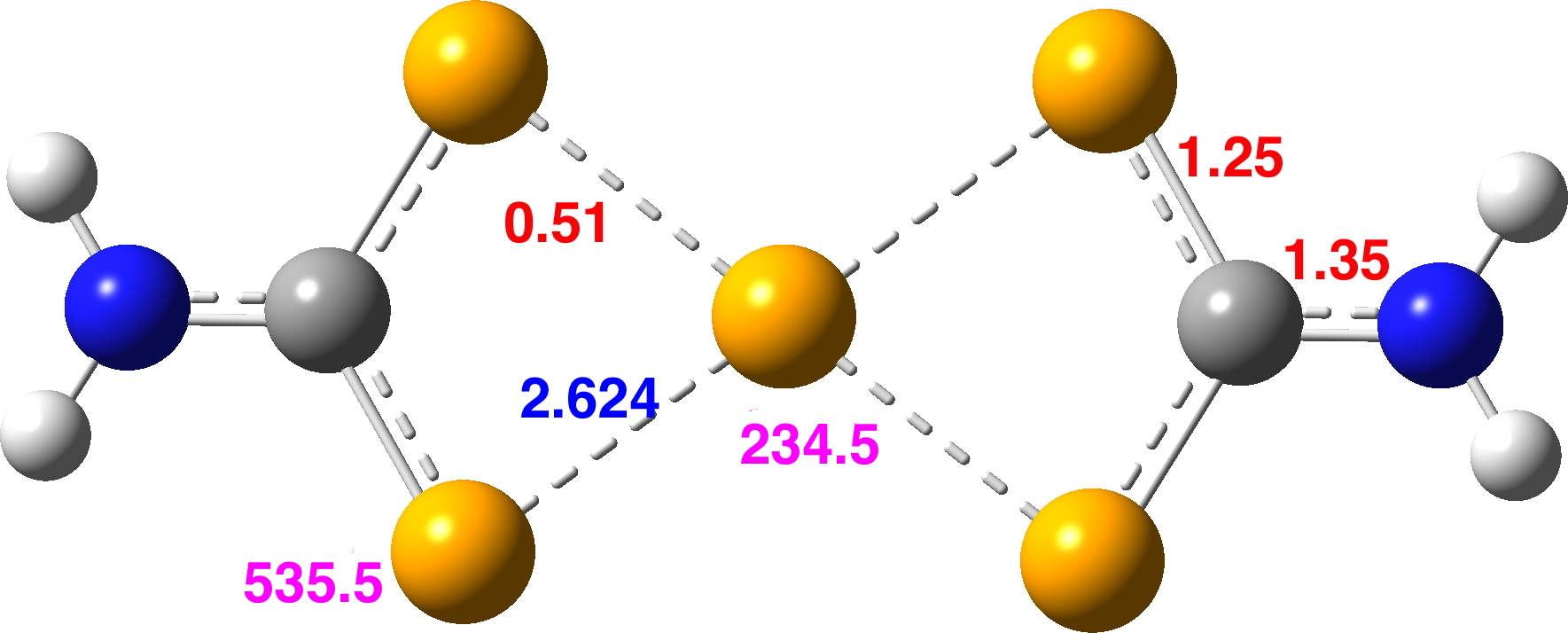The calculated 77Se shifts are shown in magenta, with the observed values being 655 and 255 ppm. The match is not good, the errors were 120 and 20.5 ppm.  However calculated shifts for elements adjacent to e.g. Se or Br etc suffer from relativistic effects such as spin orbit coupling. Thus the shift for the central Se, surrounded by four other Se atoms is likely to have a significant error, but the error for the four other Se atoms should be less. The reverse is true.

However, all the calculations of this species (up to Def2-TZVPPD basis set) showed this symmetric form of D2h symmetry to actually be a transition state, as per below.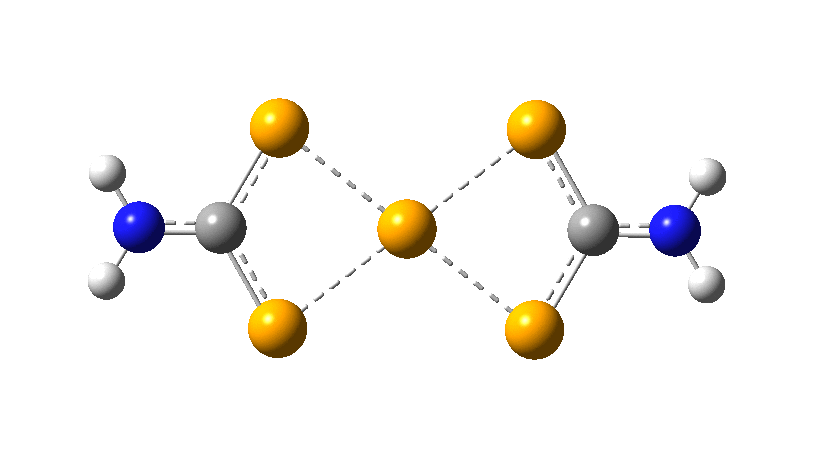There is a minimum with the structure below in which one pair of Se-Se lengths are longer than the other pair and for which the free energy is 6.5 kcal/mol lower. The Wiberg bond orders for the two sets of Se-Se bonds are now 0.16 and 0.86, which very much corresponds to structure 3 above.Assuming that this compound is fluxional even at -50°C, the average of the pairs of Se atoms gives calculated shifts of 667 ppm (655 obs) whilst the central Se is 204.6 ppm (251 obs). The latter, influenced by two especially short Se-Se distances, is likely to have a very large spin-orbit coupling error, whilst for the former the error will be smaller (13C shifts adjacent to one Br typically have induced calculated errors of about 14 ppm).

At this point I searched the Cambridge structure database for Se coordinated by four other Se atoms. A close analogue has the structure shown below, in which pairs of Se-Se interactions have unequal bond lengths, the shorter being ~2.45Å. This matches the calculation above reasonably well.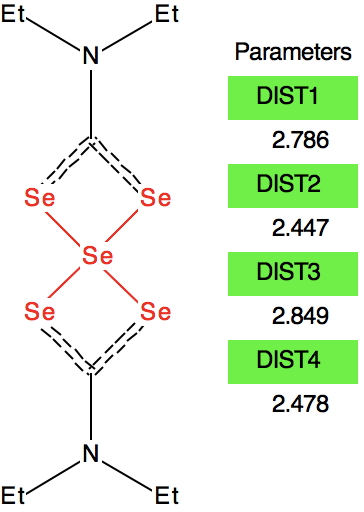Reconciling these various observations, we might assume that even at -50°C the fluxional behaviour has not been frozen out. Given that the fluxional barrier is only 6.5 kcal/mol, it is unlikely that the spectrum could be measured at a sufficiently low temperature to reveal not two sets of Se signals in the ratio 4:1 but three in the ratio 2:2:1. The spin-spin couplings reported presumably are a result of averaging a genuine 1JSe-Se coupling with a through space coupling.

So it appears that the analysis of the 77Se NMR reported in this article  may not be quite what it seems. A better interpretation is that structure 3 is the most realistic. This means no hypercoordination for the Se, never mind hypervalence!

FAIR data at DOI: 10.14469/hpc/3724. The original reference, Me2Se was incorrectly calculated without solvation by chloroform. The values shown here are now corrected from those shown in the original post.

## References

1. Y. Mazaki, and K. Kobayashi, "Structure and intramolecular dynamics of bis(diisobutylselenocarbamoyl) triselenide as identified in solution by the 77Se-NMR spectroscopy", Tetrahedron Letters, vol. 30, pp. 2813-2816, 1989. http://dx.doi.org/10.1016/S0040-4039(00)99132-9
2. D.C. Braddock, and H.S. Rzepa, "Structural Reassignment of Obtusallenes V, VI, and VII by GIAO-Based Density Functional Prediction", Journal of Natural Products, vol. 71, pp. 728-730, 2008. http://dx.doi.org/10.1021/np0705918
3. R.O. Gould, C.L. Jones, W.J. Savage, and T.A. Stephenson, "Crystal and molecular structure of bis(NN-diethyldiselenocarbamato)-selenium(II)", Journal of the Chemical Society, Dalton Transactions, pp. 908, 1976. http://dx.doi.org/10.1039/DT9760000908

### 3 Responses to “Hypervalent or not? A fluxional triselenide.”

1.Henry Rzepa says:

Here is another crystal structure with the same motif as above; RAKLOF (DOI: 10.5517/cc8mwpf). To quote the abstract of the article (See DataDOI for full details), these complexes consist of discrete, monomeric molecules featuring MSe4 cores (M=Se) that comprise true square-planar geometries..

A calculation however shows the same distortion as above to convert a true square-planar geometry to an open Se chain.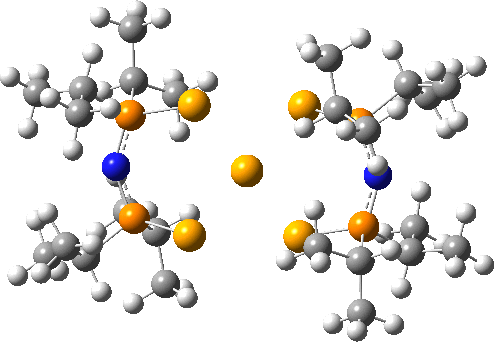You might ask how crystallography can mislead? Well, if the barrier to this distortion is low, and not constrained from happening by crystal packing, then the measured crystal coordinates will be only average positions, which are indeed square planar. It might be worth looking at this structure at lower temperatures than the room temperature that the data was collected at to see if this distortion can be detected in eg the thermal ellipsoids.

2.Henry Rzepa says:

Here are two more crystal structures, FOXNEL (DOI: 10.1002/zaac.19915920103) and SOMWIA that contain the Se112- dianion.The reported four bond lengths at the central Se for the first of these are all 2.665Å (data recorded at 140K). With the second, asymmetry is seen with pairs of lengths, 2.772 and 2.569Å. They differ only in the positive counterion.

ωB97XD/Def2-TZVPPD calculations show the symmetric form to be a second order saddle point, with the two asymmetric distortions as shown below (RSe-Se 2.68Å). Again, the apparent symmetry in the crystal structures may be due to the low barriers involved, coupled with crystal packing forces that might tend to symmetrise the structures. The free energy of the open chain Se11 form is 20.2 kcal/mol lower than the square planar transition state, which suggests that the crystal packing forces coupled with stabilizations due to the counter-ion must be similar in magnitude.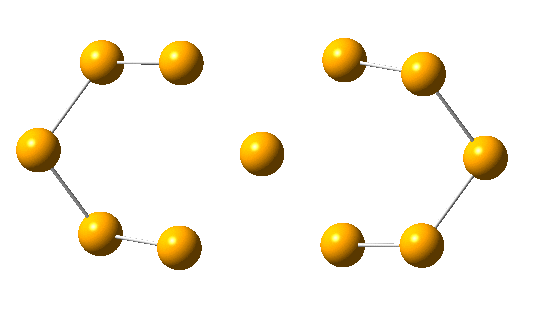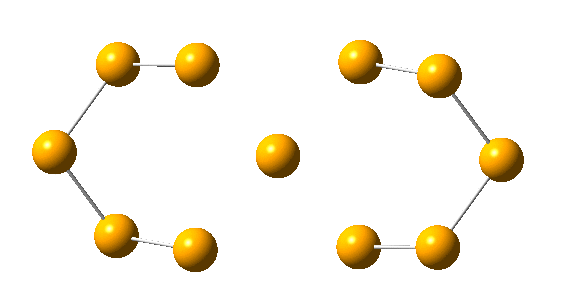3.Mark Penick says:

It’s good that you found FOXNEL and RAKLOF, because the Japanese molecule from 1989 has four-membered rings and these don’t. If the 1989 molecule fails to prove itself hypervalent, the failure could be put down to ring strain. The hypervalent interaction might not be strong enough to hold a four-membered ring closed. RAKLOF and FOXNEL don’t have this problem.